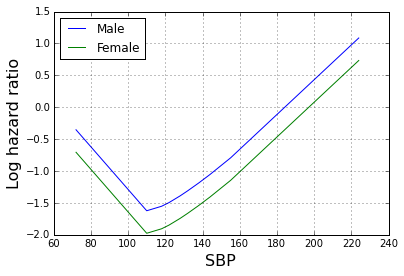Survival analysis using NHANES mortality data¶

This notebook demonstrates several methods for survival analysis that are available in Statsmodels, using the NHANES III study data.

In :
import statsmodels
import numpy as np
import pandas as pd
import statsmodels.api as sm
import os

import matplotlib.pyplot as plt
%matplotlib inline

NHANES is a cross sectional study (subjects are assessed at one time point and are never re-assessed by NHANES). The NHANES study team periodically uses social security and other sources of death records to determine which study subjects are still alive at a given follow up point. Here we will use the mortality data from 2011. People who are died prior to 2011 should have a date of death in these mortality files. People who are alive in 2011 will be considered to be censored in the survival analyses.

The mortality data are available at the link below as a fixed width format (FWF) file. The file to retrieve is called "NHANES_III_MORT_2011_PUBLIC.dat".

We downloaded the mortality data and gzipped it. To read a FWF file we need a specification defining which values appear in each column of the data file. This is contained in the SAS script provided with the data:

Copy lines 119 to 141 from the SAS program and place it into a text file called "mortality_fields.txt". The following utility function "get_colspecs" will read this file and use it to produce a list of start/end points for the pandas read_fwf function to use when reading the mortality data file.

In :
def get_colspecs(fname):
fields = []
fid = open(fname)
columns = []
colspecs = []
for line in fid:
v = line.rstrip().split()
columns.append(v)
u = v.split("-")
if len(u) == 1:
u = [int(u), int(u)]
else:
u = [int(u), int(u)]
u -= 1
colspecs.append(u)
return columns, colspecs

In :
columns, colspecs = get_colspecs("mortality_fields.txt")
fname = "NHANES_III_MORT_2011_PUBLIC.dat.gz"
compression="gzip")

The data dictionary for the mortality data is here:

The only variables we will use here are "PERMTH_INT", which is the time duration from the NHANES clinical assessment to the last follow up time, and "MORTSTAT", which indicates whether the follow up interval ends with death or censoring. We will also need the "SEQN" variable to erge the mortality and assessment data. Therefore we wil drop the other columns, and drop the cases with missing data on these key variables.

In :
mort = mort[["MORTSTAT", "PERMTH_INT", "SEQN"]].dropna()

We will also need the data from the NHANES 3 assessment. The data and codebooks are available here:

http://www.cdc.gov/nchs/nhanes/nh3data.htm

In the analyses below we will use the adult data. As with the mortality data considered above, this is a fixed-width format file. The column specifications are given in the SAS file that can be downloaded from the above link.

In :
compression="gzip")

Now we merge the mortality and visit data.

In :
df = pd.merge(data, mort, left_on="SEQN", right_on="SEQN")
print(mort.shape)
print(data.shape)
print(df.shape)
(19592, 3)
(20050, 1238)
(19592, 1240)

Next we drop the columns that we will not use below, and drop cases with missing data.

In :
df = df[["PERMTH_INT", "MORTSTAT", "HSAGEIR", "HSSEX", "HAZA8AK1", "SEQN"]].dropna()

Just for orientation, a histogram of age values is shown below.

In :
plt.hist(df.HSAGEIR, color='grey')
plt.xlabel("Age (years)", size=16)
plt.ylabel("Frequency", size=16)
Out:
<matplotlib.text.Text at 0x7f1b1645fa90>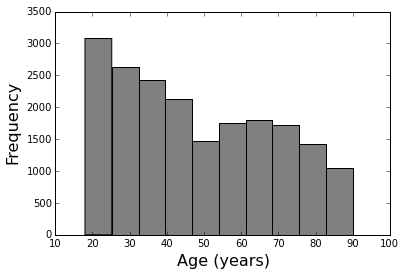Estimates of the survival function¶

We can now create a survival function object. To do this we need to provide the duration values ("PERMTH_INT") and the mortality status variable ("MORTSTAT"). The "summary" method of the survival function returns a dataframe containing the Kaplan-Meier estimates of the survival function at its steps. For any survival analysis it is important to define time zero in a meaningful way. There are various ways to do this here, but one approach suitable here is to select people in a narrow age range, say 50-55. This means we are looking at the survival function for one "birth cohort" -- people who were roughly age 52.5 when the NHANES III assessmsents took place.

In :
ii = (df.HSAGEIR >= 50) & (df.HSAGEIR < 55)
df = df.loc[ii]
In :
sf1 = sm.duration.SurvfuncRight(df["PERMTH_INT"], df["MORTSTAT"], "")
Out:
Surv prob Surv prob SE num at risk num events
Time
0 0.999044 0.000956 1046 1
1 0.998088 0.001351 1045 1
5 0.996176 0.001908 1044 2
6 0.995220 0.002133 1042 1
7 0.993308 0.002521 1041 2

The Kaplan-Meier estimate is the most common estimator of the survival function. We plot that next. The "+" signs indicate censoring. Since NHANES III took place in the 1980's, all subjects have at least 12 years of follow-up as of 2011.

In :
sf1.plot()
plt.grid(True)
plt.ylim(0.6, 1)
plt.xlabel("Time (months)", size=16)
plt.ylabel("Proportion alive", size=16)
Out:
<matplotlib.text.Text at 0x7f1b035399b0>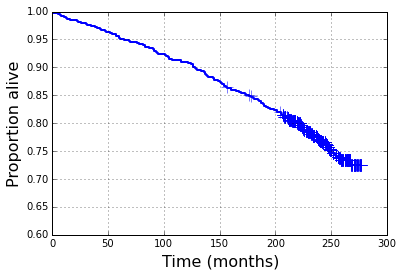Next we plot the survival function together with point-wise standard errors. . Since the NHANES sample size is large, the standard errors are small. This means that we have estimated the survival function quite precisely.

In :
plt.grid(True)
plt.fill_between(sf1.surv_times, sf1.surv_prob - 2*sf1.surv_prob_se, sf1.surv_prob + 2*sf1.surv_prob_se, color="lightgrey")
plt.plot(sf1.surv_times, sf1.surv_prob, '-', color='black')
plt.ylim(0.7, 1)
plt.xlabel("Time (months)", size=16)
plt.ylabel("Proportion alive", size=16)
Out:
<matplotlib.text.Text at 0x7f1b07b7d6d8>
/usr/lib/python3/dist-packages/matplotlib/collections.py:571: FutureWarning: elementwise comparison failed; returning scalar instead, but in the future will perform elementwise comparison
if self._edgecolors == str('face'):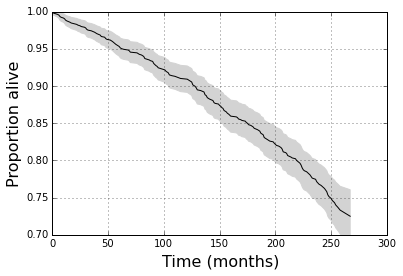A more rigorous way to assess the uncertainty in the survival function estimate is to use simultaneous confidence bands rather than pointwise confidence bands. There are several methods in the literature for doing this. The standard methods do not give very meaningful results for survival times close to the extreme values of oberved durations (0 and 270 here).

In :
lcb, ucb = sf1.simultaneous_cb(transform='arcsin')
plt.grid(True)
plt.plot(sf1.surv_times, sf1.surv_prob, '-', color='black')
plt.fill_between(sf1.surv_times, lcb, ucb, color='lightgrey')
Out:
<matplotlib.collections.PolyCollection at 0x7f1b03eb3390>
/usr/lib/python3/dist-packages/matplotlib/collections.py:571: FutureWarning: elementwise comparison failed; returning scalar instead, but in the future will perform elementwise comparison
if self._edgecolors == str('face'):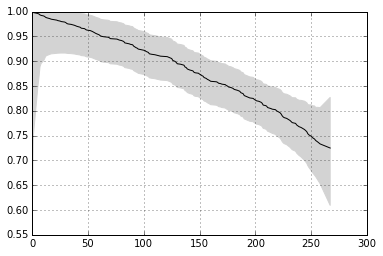Survival function quantiles¶

The inverse of the survival function is the survival quantile function. It tells us the survival time corresponding to each probability point (e.g. what is the 10th percentile of survival times). These estimates may be undefined for probabiity points that are "not reached". For example, in the overall NHANES sample the median survival time is not reached.

The first plot below shows the survival quantles up to the 20th percentile, which is the highest probability point that is reached in these data.

In :
qp = np.linspace(0.01, 0.19, 20)
sq = [sf1.quantile(x) for x in qp]

plt.clf()
plt.grid(True)
plt.plot(qp, sq, '-')
plt.xlabel("Probability", size=16)
plt.ylabel("Survival quantile", size=16)
Out:
<matplotlib.text.Text at 0x7f1b03e787f0>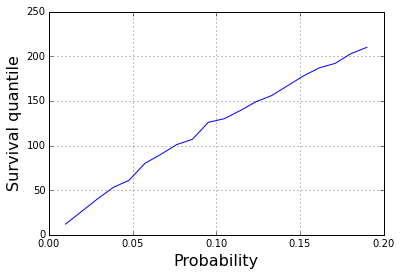We can put pointwise confidence bands around the survival quantile curves.

In :
ci = [sf1.quantile_ci(x) for x in qp]
ci = np.asarray(ci)

plt.clf()
plt.grid(True)
plt.fill_between(qp, ci[:, 0], ci[:, 1], color='lightgrey')
plt.plot(qp, sq, '-', color='black')
plt.xlabel("Probability", size=16)
plt.ylabel("Survival quantile", size=16)
Out:
<matplotlib.text.Text at 0x7f1b05e9ac88>
/usr/lib/python3/dist-packages/matplotlib/collections.py:571: FutureWarning: elementwise comparison failed; returning scalar instead, but in the future will perform elementwise comparison
if self._edgecolors == str('face'):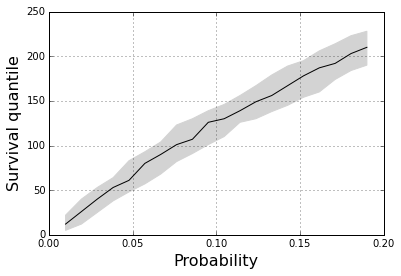Group-wise comparisons of survival functions¶

To illustrate the methods for comparing groups, we will compare survival curves for women and men. The gender information is in the "HSSEX" variable. HSSEX=1 indicates males and HSSEX=2 indicates females.

In :
ii = (df.HSSEX==1)
sfm = sm.duration.SurvfuncRight(df.loc[ii, "PERMTH_INT"], df.loc[ii, "MORTSTAT"], "Male")

ii = (df.HSSEX==2)
sff = sm.duration.SurvfuncRight(df.loc[ii, "PERMTH_INT"], df.loc[ii, "MORTSTAT"], "Female")

We can now plot the survival function estimates for males and females (in the 50-60 age range) on the same axes.

In :
ax = plt.gca()

plt.grid(True)
sfm.plot(ax)
sff.plot(ax)
plt.xlabel("Time (months)", size=16)
plt.ylabel("Proportion alive", size=16)
plt.ylim(0.5, 1)
ha, lb = ax.get_legend_handles_labels()
plt.legend(ha, lb, loc="lower left")
Out:
<matplotlib.legend.Legend at 0x7f1b05e9a8d0>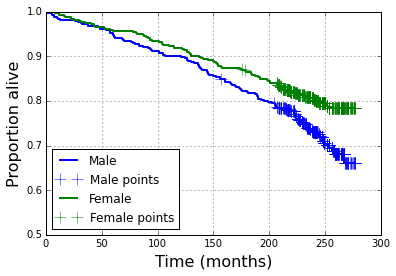In :
chisq, pval = sm.duration.survdiff(df.PERMTH_INT, df.MORTSTAT, df.HSSEX)
print(chisq, pval)
8.3362343064 0.00388620655464

Proportional hazards regression analysis¶

To move beyond group-wise comparisons, we can use regression analysis. There are several methods of regression analysis that are suitable for data with censoring. The most commonly used in practice is the proportional hazards regression model, or "Cox model". Below we run a very simple proportional hazards regression model using gender as a predictor of mortality.

In :
model1 = sm.PHReg(df.PERMTH_INT, df.HSSEX, status=df.MORTSTAT)
result1 = model1.fit()
result1.summary()
Out:
 Model: PH Reg Sample size: 1046 Dependent variable: PERMTH_INT Num. events: 246 Ties: Breslow
log HR log HR SE HR t P>|t| [0.025 0.975] -0.3678 0.1282 0.6923 -2.8693 0.0041 0.5385 0.8900

Next we will fit some models including blood pressure as a predictor of mortality. The blood pressure variable has a special code 888 indicating missing values. Below we remove all records with these values from the data set, and check the distribution of the remaining values.

In :
ii = (df.HAZA8AK1 != 888)
df = df.loc[ii]
plt.hist(df.HAZA8AK1.values)
plt.xlabel("SBP", size=16)
plt.ylabel("Frequency", size=16)
Out:
<matplotlib.text.Text at 0x7f1b09376278>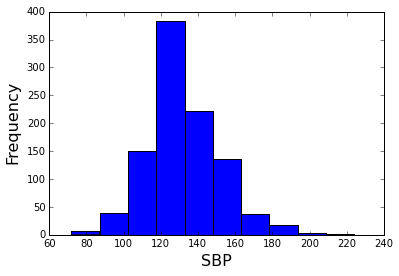Next we use a formula to fit a regression model including main effects and interactions for blood pressure and sex. Note that the proportional hazards regression models must not have an intercept, so we add "0 +" to the formula.

In :
model2 = sm.PHReg.from_formula("PERMTH_INT ~ 0 + HAZA8AK1*HSSEX", status="MORTSTAT", data=df)
result2 = model2.fit()
result2.summary()
Out:
 Model: PH Reg Sample size: 995 Dependent variable: PERMTH_INT Num. events: 231 Ties: Breslow
log HR log HR SE HR t P>|t| [0.025 0.975] 0.0216 0.0100 1.0218 2.1604 0.0307 1.0020 1.0421 -0.2086 0.8994 0.8117 -0.2319 0.8166 0.1393 4.7309 -0.0009 0.0064 0.9991 -0.1344 0.8931 0.9866 1.0118

Since the blood pressure effect may not be linear, we can try modeling it using splines.

In :
model3 = sm.PHReg.from_formula("PERMTH_INT ~ 0 + bs(HAZA8AK1, 4) + HSSEX", status="MORTSTAT", data=df)
result3 = model3.fit()
result3.summary()
Out:
 Model: PH Reg Sample size: 995 Dependent variable: PERMTH_INT Num. events: 231 Ties: Breslow
log HR log HR SE HR t P>|t| [0.025 0.975] -1.6206 1.2091 0.1978 -1.3403 0.1801 0.0185 2.1153 -0.9778 1.0216 0.3761 -0.9571 0.3385 0.0508 2.7859 0.5770 1.3702 1.7807 0.4211 0.6737 0.1214 26.1183 1.4392 1.6687 4.2175 0.8625 0.3884 0.1602 111.0392 -0.3530 0.1335 0.7026 -2.6437 0.0082 0.5408 0.9127

We can use the predict_functional function to graph the relationship between blood pressure and the mortality hazard. The association is U-shaped -- people with very low or very high blood pressure are both at higher risk of death. The risk of death for people with low blood pressure could be due to confounding with other factors that induce both low blood pressure and death.

In :
from statsmodels.sandbox.predict_functional import predict_functional

plt.clf()
plt.grid(True)

sexl = {1: "Male", 2: "Female"}

for sex in 1,2:
pr, cb, fv = predict_functional(result3, "HAZA8AK1", values={"HSSEX": sex})
plt.plot(fv, pr.predicted_values, label=sexl[sex])

ha, lb = plt.gca().get_legend_handles_labels()
plt.legend(ha, lb, loc="upper left")

plt.xlabel("SBP", size=16)
plt.ylabel("Log hazard ratio", size=16)
/projects/3a549bb7-8b7c-41a0-83e7-b37fd3da53a0/statsmodels-master/statsmodels/sandbox/predict_functional.py:169: UserWarning: 'MORTSTAT', 'SEQN', 'HSAGEIR' in data frame but not in summaries or values.
% ", ".join(["'%s'" % x for x in unmatched]))
Out:
<matplotlib.text.Text at 0x7f1b0b6ca6d8>